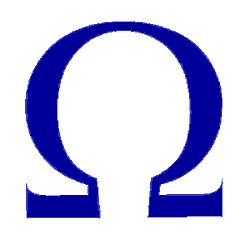Electronics – Electrical Unit Definitions

Definitions of basic measurement units in electricity and electronics

Ampere (A)

Unit of measurement of electric current. Is the amount of charge that flows through a conductor in a unit of time. I = Q/t

Ampere is the electric current (I) that produces a force of 2 x 10-7newton per meter between two parallel conductors separated by 1 meter. 1 A = 1 coulomb / second. 1 A = 1000 mA (milliampere). See also:Coulomb (C)

Coulomb is the measuring unit of the electric charge. Q load passing through a point in one second when the current is 1 ampere. 1 Coulomb = 6.28×1018 electrons.
See: Coulomb’s Law

Joule (J)

Joule is the work (W) made by the force of one Newton acting over the distance of 1 meter.

Watt (W)

Watt is the power unit. Power (P) required to perform a work at a rate of 1 joule per second.
See: Joule’s Law

Farad is the capacitance measuring unit. Farad is the capacitance (C) where the load of 1 coulomb produces a voltage of 1 volt. See also:

Henry (H)

Henry is the inductance measurement unit. Henry is the inductance (L) where 1 volt is induced by a change of 1 ampere per second. See also:

Ohm (Ω)

Unit of measurement of the electrical resistance, represented by the capital Greek letter (Ω) omega. Ohm is the resistance that produces a voltage of 1 volt when it is traversed by a current of 1 ampere. See also:

• Electrical resistance
• Variable resistor (potentiometer, rheostat)
• Wirewound resistor
• Resistors color coding
• Measuring ranges for resistors on a VOM
• Measure resistance of low value
• Measure sensitive resistors

Siemens (S)

Siemens is the unit of measure of the conductance (G). Siemens is the conductance which produces a current of 1 ampere when a voltage of 1 volt is applied. It is the reciprocal of the ohm, formerly called mho.

Volt (V)

Volt is the unit measuring the electrical potential difference or voltage. Voltage is the potential difference between two points in a conductor carrying a current of 1 ampere when the power dissipated between the two points is 1 watt.

Hertz (Hz)

Hertz is the number of complete cycles of a wave in a unit of time. 1 Hertz = 1 cycle/sec. See also: Alternating Current (AC)

Radian is the angle which covers the circumference portion which is equal to the length of the radius of the circle.

Angular frequency (w)

Angular frequency is radians per second. w = 2nf. (n = pi). see also: What is a radian? – Angular frequency

π = 3.14159265359….

Time (t)

Time measurement unit (Sec) See: Time constant.

•
•
•
•
•
•
•
•
•
•
•
•
•
•
•
•
•
•